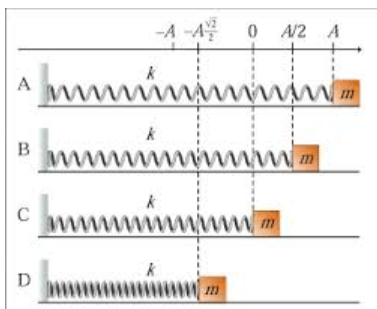# Problem: Figure 1 shows a harmonic oscillator at four different moments, labeled A, B, C, and D. Assume that the force constant k, the mass of the block, m, and the amplitude of vibrations, A, are given. We will also assume that there are no resistive forces so the total energy of the oscillator remains constant.What is the total energy of the system?

###### FREE Expert Solution

Spring potential energy:

$\overline{){\mathbf{U}}{\mathbf{=}}\frac{\mathbf{1}}{\mathbf{2}}{\mathbf{k}}{{\mathbf{x}}}^{{\mathbf{2}}}}$

Total energy:

E = U + KE

91% (373 ratings)###### Problem Details

Figure 1 shows a harmonic oscillator at four different moments, labeled A, B, C, and D.Assume that the force constant k, the mass of the block, m, and the amplitude of vibrations, A, are given. We will also assume that there are no resistive forces so the total energy of the oscillator remains constant.

What is the total energy of the system?